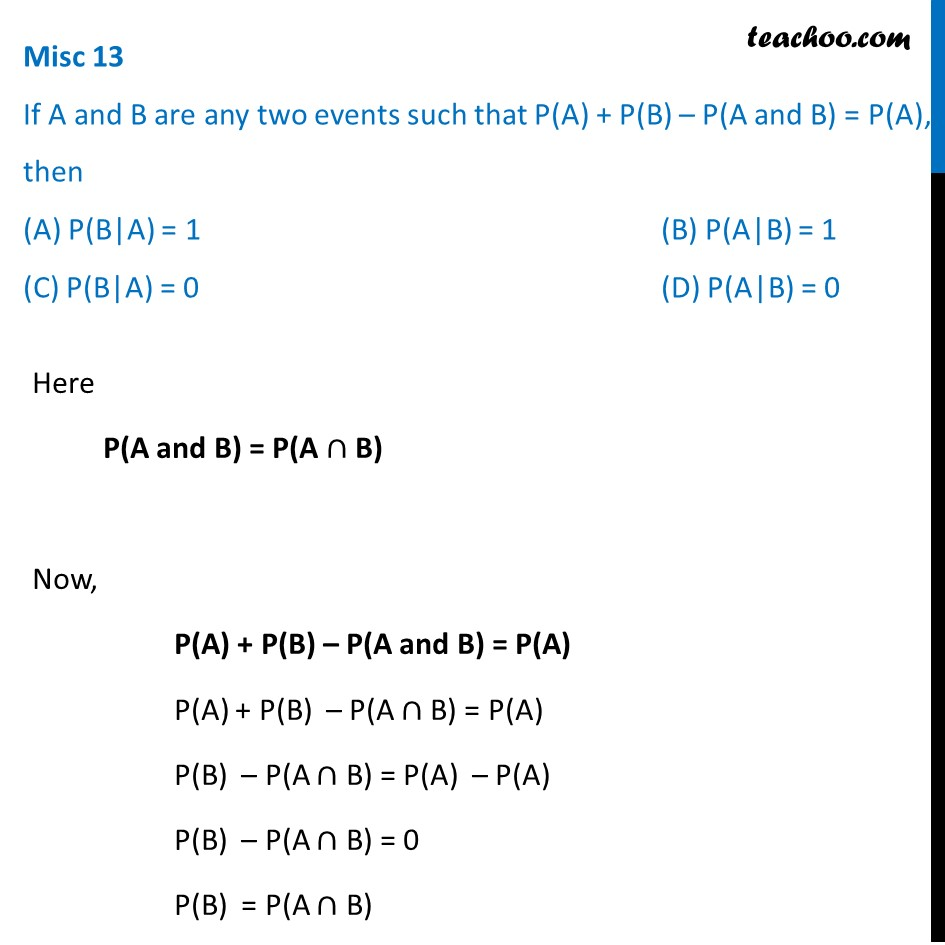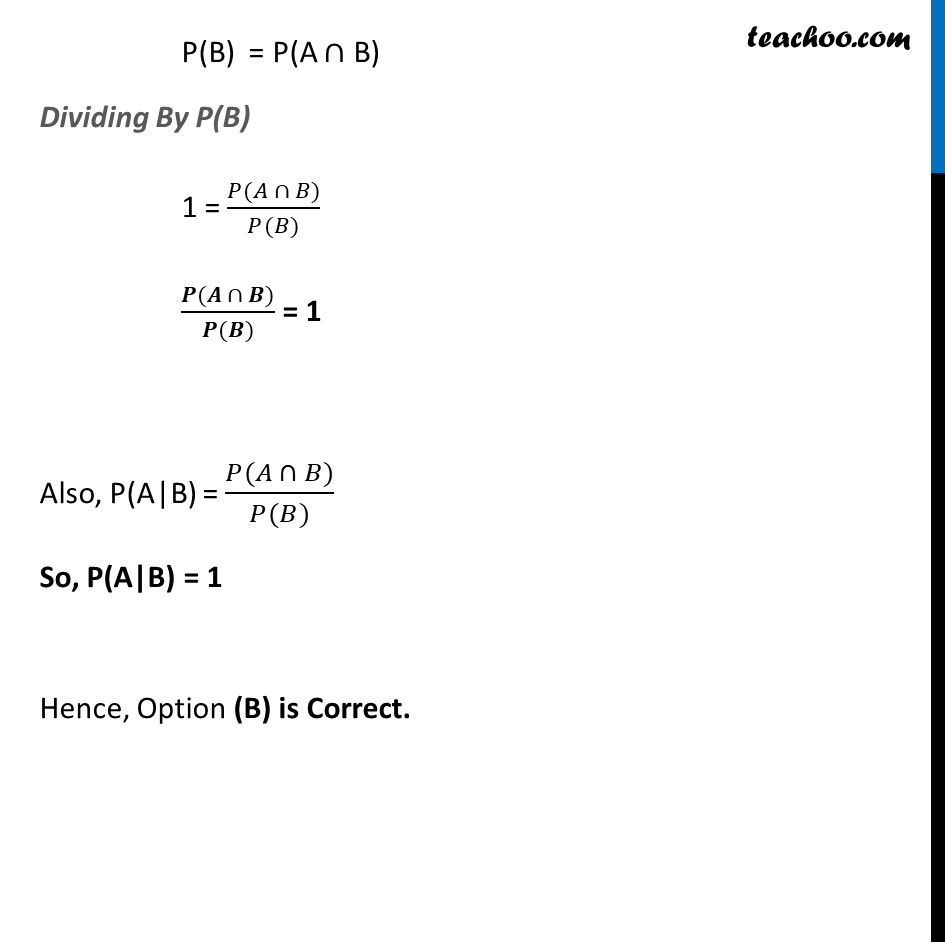Miscellaneous

Chapter 13 Class 12 Probability
Serial order wiseLearn in your speed, with individual attention - Teachoo Maths 1-on-1 Class

### Transcript

Misc 13 If A and B are any two events such that P(A) + P(B) – P(A and B) = P(A), then (A) P(B|A) = 1 (B) P(A|B) = 1 (C) P(B|A) = 0 (D) P(A|B) = 0 Here P(A and B) = P(A ∩ B) Now, P(A) + P(B) – P(A and B) = P(A) P(A) + P(B) – P(A ∩ B) = P(A) P(B) – P(A ∩ B) = P(A) – P(A) P(B) – P(A ∩ B) = 0 P(B) = P(A ∩ B) P(B) = P(A ∩ B) Dividing By P(B) 1 = (𝑃(𝐴 ∩ 𝐵))/(𝑃(𝐵)) (𝑷(𝑨 ∩ 𝑩))/(𝑷(𝑩)) = 1 Also, P(A|B) = (𝑃(𝐴 ∩ 𝐵))/(𝑃(𝐵)) So, P(A|B) = 1 Hence, Option (B) is Correct.